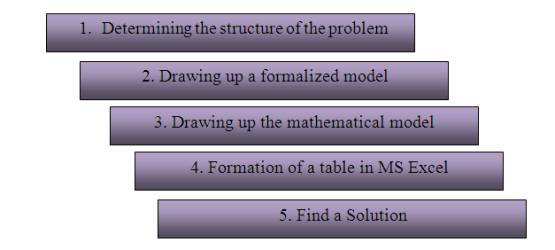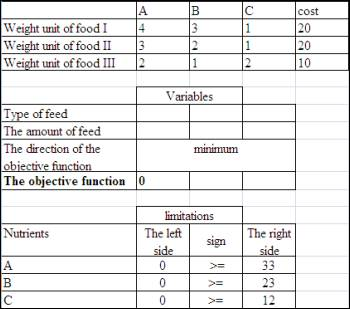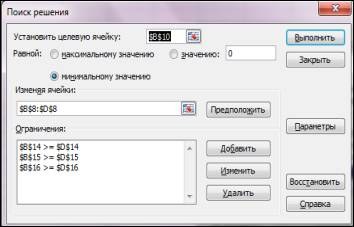Экономические науки/8. Математические методы в экономике

Serkebayeva L.T

A.Baitursynov Kostanai State University, Kazakhstan

Technology for solving optimization problems.

In solving the economic problems often raises the question of choosing the best option solutions. In other words, we want to solve the optimization problem, which consists in choosing the best solution among many possible options.

The optimization can be achieved by setting the mathematical modeling of economic and social systems. The optimization problem can be formulated in the language of mathematics: many available options are described using mathematical relationships (equalities, inequalities, equations) and each decision is assessed quantitatively using the indicator. This figure is called the objective function. Then the best solution is the one that delivers the objective function maximum or minimum value. The direction of the objective function depends on the meaning of the problem.

Typical problems of optimization and management decisions implemented by using the tool "Search for Solutions" in MS Excel have three main properties:

-     there is only one target to optimize;

-     there are limitations recorded in the form of inequalities;

-     a set of input values.

Options are limited to those limit values:

-          the number of unknowns - 200;

-          the number of restrictions on the unknown – 100;

-          number of conditions on the unknown - 400.

The algorithm for finding optimal solutions includes several stages:

-          preparatory work;

-          debugging solutions;

-          analysis solutions.

The sequence of preparatory work carried out in solving economic and mathematical modeling using MS Excel is shown in Figure 1.Figure 1. The scheme of the preparatory work

Let us discuss in detail in paragraphs 4 and 5 of the scheme. Let consider the following problem: For feeding the farm animals in their daily diet should include at least 33 units of nutrient A, 23 units of substance B and 12 units substance С. For feed use 3 types of feed. Content information nutrients and unit cost of each feed are given in Table 1.

Table 1

 А В С cost Weight unit of food I 4 3 1 20 Weight unit of food II 3 2 1 20 Weight unit of food III 2 1 2 10

It takes up the least expensive diet in which every animal would get the necessary nutrients A, B, C.

Adding data to a spreadsheet for further solutions using the tool "Search for solutions" can be adjusted as follows (Figure 2).

By the "Data - Search solutions" to open the window "Search for Solutions" (Figure 3).

After obtaining the optimal solution is carried out its analysis.

The analysis of the optimal solution is the final stage of mathematical modeling of economic processes.Figure 2. Making the data in the table MS Excel.Figure 3. Filling the tool window " Search for Solutions"

It allows for a more accurate check of conformity to the model of economic processes.  Вut it does not exclude or replace the traditional analysis of the plan from the economic perspective before making management decisions.

Literature:

1.   В.Н.Пакулин. Решение задач оптимизации управления в MS Excel 2010

2.   Тарасенко Н.В., Шеломенцева Н.Н. Математика-2. Решение задач оптимизации в Excel: Метод. указ. для студентов экономических специальностей. – Иркутск: Изд-во БГУЭП, 2003. – 43с.